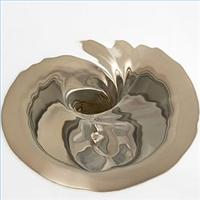# sandbox/Antoonvh/bathtub.cA vortex may appear when you drain your bath.

# A bathtub vortex

A cylindrdical water-filled sink with water depth h and radius R is drained via a centered opening in the bottom (radius r), via the acceleration of gravity (g). With the properties of water (\rho_w, \mu_w) and air (\rho_a, \mu_a), one may identify the following dimensionless groups:

\displaystyle \Pi_1 = \frac{\rho_w}{\rho_a}, \displaystyle \Pi_2 = \frac{\mu_w}{\mu_a}, \displaystyle \Pi_3 = \frac{R}{r}, \displaystyle \Pi_4 = \sqrt{\frac{\rho_w^2gr^3}{\mu_w^2}}. \displaystyle \Pi_5 = \frac{h}{r}

For a typical sink-drain-scenario, values are: \Pi_1 = 1000, \Pi_2 = 50, \Pi_3 = 32 and \Pi_4 = 3000, \Pi_5 = 3.

Furtheremore, when the fluid is initially rotating with anglular frequency \Omega, a sixth number arises:

\displaystyle \Pi_6 = \frac{g}{r\Omega^2} \approx 6400.

In our setup, we use unitary values for r, g and \rho_w and as such, \Omega = 0.0128, \mu_w = 0.02, R = 32, \mu_a = 0.0004, \rho_a = 0.001. and h = 10.

#include "axi.h"
#include "navier-stokes/centered.h"
#include "navier-stokes/swirl.h"
#include "two-phase.h"
#include "view.h"
#include "particles.h"

face vector av[];
double Omega = 0.0125;
double h = 3;
double RD = 32;

int main(){
mu1 = 0.02;
mu2 = 0.0004;
rho2 = 0.001;
rho1 = 1.;
L0 = 2*RD;
N = (int)(L0 + 0.5);
a = av;
run();
}

We use mask() to implement the bath geometry.

bid tub;
u.n[tub] = dirichlet(0);

event init (t = 0) {
mask(x - RD  < 0 && x - RD > -2  && y < RD && y > 1 ? tub : none);
mask(y - RD > 0 && y - RD < 2 && fabs(x - RD - 4) < 6. ? tub : none);
foreach() {
if (y < RD && x > RD && x < RD + h)
f[] = 1;
}
foreach()
w[] = y*Omega*f[];
refine (level < 7);
DT = 0.01;

For visualization, we add tracer particles.

  int nx = 5, ny = 30;
n_part = nx*ny;
loc = malloc (sizeof(coord)*n_part);
int ii = 0;
for (int j = 0; j < nx ; j++){
for (int k = 0; k < ny; k++){
loc[ii].x = RD + 0.02 + 0.9*(double)j*(h/((double)nx - 1.));
loc[ii].y = 0.02 + 0.95*(double)k*(RD/((double)ny - 1.));
loc[ii].z = noise()*pi;
ii++;
}
}
}

int j = 0;
while (j < n_part){
loc[j].z += dt* (interpolate(w, loc[j].x, loc[j].y))/loc[j].y;
j++;
}
}

event acceleration (i++) {
coord n = {-1, 0};
foreach_face()
av.x[] = n.x;
}

The water that has been drained is removed from the simulation.

event remove_f (i++){
foreach(){
if (x < RD - 2*h)
f[] = 0;
}
}

adapt_wavelet ({u.x, u.y, w, f}, (double[]){0.01, 0.01, 0.01, 0.01}, 8, 6);

event stop (t = 100);

## Result

We generate the resulting movie below. It shows the azimutal velocity (left) and the water (right), together with the tracer particles.

We see the emergence of a air-filled vortex core.

static void glvertex3d (bview * view, double x, double y, double z) {
if (view->map) {
coord p = {x, y, z};
view->map (&p);
glVertex3d (p.x, p.y, p.z);
}
else
glVertex3d (x, y, z);
}

struct _scatter_axi {
coord * loc;    // Coordinates
float s, pc, coefs; // point size, colour and distance attenuation coefs.
};

void glPointParameterfv (GLenum pname, const GLfloat * params);

trace
void scatter_axi (struct _scatter_axi p){// Modified from scatter.h
bview * view = draw();
if (!p.coefs){ // A guess:
p.coefs = 0.01;
p.coefs = 0.2;
p.coefs = 0.5;
}
glPointParameterfv (GL_POINT_DISTANCE_ATTENUATION, p.coefs);
if (p.pc)
glColor3f(p.pc, p.pc, p.pc);
if (!p.s)
p.s = 20;
glPointSize(p.s);
glBegin (GL_POINTS);
for (int j = 0; j < n_part; j++)
glvertex3d (view, p.loc[j].x, p.loc[j].y*cos(loc[j].z), p.loc[j].y*sin(loc[j].z));
glEnd();
view->ni++;
}

event mov(t += 0.1){
view (fov = 6, theta = 0.2, psi = -pi/2.,
ty = -0.5, width = 600, height = 150);
squares ("w");
draw_vof ("f");
scatter_axi (loc = loc);
mirror({0,-1})
draw_vof ("f", filled = 1, fc = {0.1, 0.1, 0.8});
save ("movie.mp4")
}